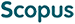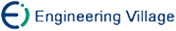廖士中, 王 梅, 赵志辉. 正定矩阵支持向量机正则化路径算法[J]. 计算机研究与发展, 2013, 50(11): 2253-2261.
 引用本文: 廖士中, 王 梅, 赵志辉. 正定矩阵支持向量机正则化路径算法[J]. 计算机研究与发展, 2013, 50(11): 2253-2261.Liao Shizhong, Wang Mei, Zhao Zhihui. Regularization Path Algorithm of SVM via Positive Definite Matrix[J]. Journal of Computer Research and Development, 2013, 50(11): 2253-2261.
 Citation: Liao Shizhong, Wang Mei, Zhao Zhihui. Regularization Path Algorithm of SVM via Positive Definite Matrix[J]. Journal of Computer Research and Development, 2013, 50(11): 2253-2261.## Regularization Path Algorithm of SVM via Positive Definite Matrix

• 摘要: 正则化路径算法是数值求解支持向量机 (support vector machine, SVM)分类问题的有效方法，它可在相当于一次SVM求解的时间复杂度内得到所有的正则化参数及对应SVM的解.现有的SVM正则化路径算法或者不能处理具有重复数据、近似数据或线性相关数据，或者计算开销较大.针对这些问题，应用正定矩阵方程组求解方法来求解SVM正则化路径，提出正定矩阵SVM正则化路径算法(positive definite SVM path, PDSVMP).PDSVMP算法将迭代方程组的系数矩阵转换为正定矩阵，并采用Cholesky分解方法求解路径上各拐点处Lagrange乘子增量向量；与已有算法中直接求解正则化参数不同，该算法根据活动集变化情况确定参数增量，并在此基础上计算正则化参数，这样保证了理论正确性和数值稳定性，并可降低计算复杂性.实例数据集及标准数据集上的实验表明，PDSVMP算法可正确处理包含重复数据、近似数据或线性相关数据的数据集，并具有较高的计算效率.

Abstract: The regularization path algorithm is an efficient method for numerical solution to the support vector machine (SVM) classification problem, which can fit the entire path of SVM solutions for every value of the regularization parameter, with essentially the same computational cost as fitting one SVM model. Existing SVM regularization path algorithms can neither deal with the datasets having duplicate data points, nearly duplicate points, or points that are linearly dependent efficiently, nor have efficient numerical solution. To address these issues, an improved regularization path algorithm via positive definite matrix positive definite SVM path (PDSVMP) is proposed in this paper, which provides the accurate path of SVM solutions. The coefficient matrix of the system of iteration equations is transformed into a positive definite matrix, then the Lagrange multiplier increment vector is computed by Cholesky decomposition, and the increment of regularization parameter is derived according to the changes of the active set, which is used to compute the regularization parameter on each inflection point. Such treatment is able to guarantee the theoretical correctness and numerical stability, and reduce the computational complexity. Experimental results on instance dataset and benchmark datasets show that the PDSVMP algorithm can effectively and efficiently handle datasets having duplicate data points, nearly duplicate points, or points that are linearly dependent./下载:  全尺寸图片 幻灯片
• 分享
• 用微信扫码二维码

分享至好友和朋友圈